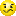# LabVIEW

cancel
Showing results for
Did you mean:

## low pass filter with formula node

Hi guys.

My teacher gave me an exercise (attached) in which i have to realize a low pass filter with the formula node. He gave me also the input/output but I  have no idea where to start.

Can you help me?

Message 1 of 7
(2,592 Views)

## Re: low pass filter with formula node

Well, we will not do your homework for you.

1. Please as a specific question. "Can you help me ?" does not tell us what kind of help you need.

2. What are the desired characteristics of the filter? Cutoff frequency? Stop band attenuation? Passband attenuation and ripple?

3. How much do you know about LabVIEW?

Lynn

Message 2 of 7
(2,589 Views)

## Re: low pass filter with formula node

Hi john well I'm quite good with the labview but I've never compared myself with a problem such this. I mean I have worked properly on array/cluster/string/save/read data but see all those data send me in panic.
I started to think on it. I have no specifics. So the cut off frequency is the one I decide.
From the theory I saw that R=1/(2*pi*ft*C) where ft=cut off frequency. So from this, since I can modify from the control the R and the C, I can obtain without problems the ft. Is it right?
Tau should be R*C is it correct?
The problem is that I have no idea what the property node are used for.
In the exercise is asked to do a control on the runtime values. Maybe this is the reason for the property node?

Message 3 of 7
(2,580 Views)

## Re: low pass filter with formula node

It sounds (from the formula you are using) as though you are using a one-pole R-C low pass filter. Tau is typically the symbol used for the time constant.

I am not sure what you mean by "do a control on the runtime values." I have not idea what the property and invoke nodes are for either.  I wondered about them when I saw your VI.

Perhaps you need to obtain clarification from the instructor.

Lynn

Message 4 of 7
(2,573 Views)

## Re: low pass filter with formula node

Well let's forget for the moment about the property node.

If for example (since i have no specifications) I want to build a Butterworth filter what should I do?

Let's do it step by step and if you can correct me.

I saw the formula for the Butterworth is:

H(jw)=1/sqrt([1+(ws/wp)^2n]) where ws= stop band freq. and wp= pass band freq.

now I know that the cutoff freq. is fc=1/(2*pi*R*C), so I suppose that ws=wc=1/R*C where R & C  are variable and choosen by the user (this is what the teacher asked).

My first obstacle is: what is wp? all the frequency?is it correct?

I am a little bit confusedMessage 5 of 7
(2,555 Views)

## Re: low pass filter with formula node

Your formula for H(jw) has no "w" in it.  The formula I usually see for a Butterworth low pass filter is |H(jw)| = 1/sqrt([1 + (w/wc)^(2*n)]) where w is the angular frequency and wc is the cutoff frequency.  For a low pass fitler you do not have stop band and pass band frequencies.

Lynn

Message 6 of 7
(2,532 Views)

## Re: low pass filter with formula node